Question

# An increase in the price level will A) shift the aggregate demand curve to the left.

7) An increase in the price level will

A) shift the aggregate demand curve to the left.

B) shift the aggregate demand curve to the right.

C) move the economy up along the aggregate demand curve.

D) move the economy down along the aggregate demand curve.

8) Expansionary monetary policy involves

A) reducing money supply and lowering taxes

B) increasing money supply to decrease interest rate

C) increasing government spending and cutting money supply

D) increasing the interest rate and increasing taxes

9) Long-run macroeconomic equilibrium occurs when

A) aggregate demand equals short-run aggregate supply.

B) structural and frictional unemployment equals zero.

C) output is at potential GDP.

D) aggregate demand equals short-run aggregate supply and they intersect at a point on the long-run

supply curve.

10) If the economy is growing beyond potential real GDP, which of the following would be an

appropriate monetary policy to bring the economy back to long-run aggregate supply? A decrease in

A) oil prices.

B) discount rate.

C) the money supply and a decrease in interest rates.

D) taxes.

Q7) option C)

Since price level changes, so no shifting of AD happens

As P rises, we move upwards along AD , bcoz Q falls, & AD slopes downwards

8) option B)

Expansionary Monetary policy occurs, when Money supply rises,

So Money Demand curve unchanged, Ms curve shifts to right , interest rate falls

9) option D)

in Long run eqm , Current gdp = potential gdp

So AD cuts SRAS , at LRAS Curve

10) option C)

As Current Output level above potential level, so follow contractionary monetray policy

So decrease Money supply, that leads to rise in interest rates ,so Investment Spending falls

Or decrease in discount rate

#### Earn Coins

Coins can be redeemed for fabulous gifts.

Similar Homework Help Questions
• ### 5) If consumption increases by \$200 and, in response, equilibrium aggregate expenditure increases by \$600, the...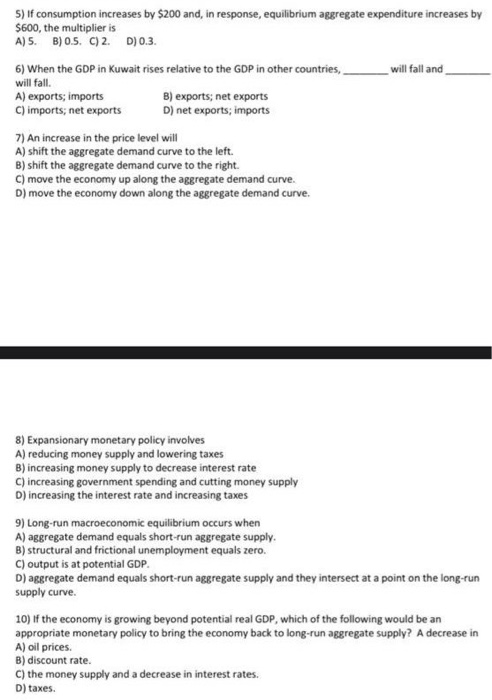5) If consumption increases by \$200 and, in response, equilibrium aggregate expenditure increases by \$600, the multiplier is A) 5 B) 0.5.C)2. D) 0.3. 6) When the GDP in Kuwait rises relative to the GDP in other countries, will fall and will fall A) exports; imports B) exports; net exports C) imports; net exports D) net exports; imports 7) An increase in the price level will A) shift the aggregate demand curve to the left. B) shift the aggregate demand...

• ### Macroeconomic Multiple Choice Questions Answer All 10 Questions* 1) If the Central Bank of Kuwait puts...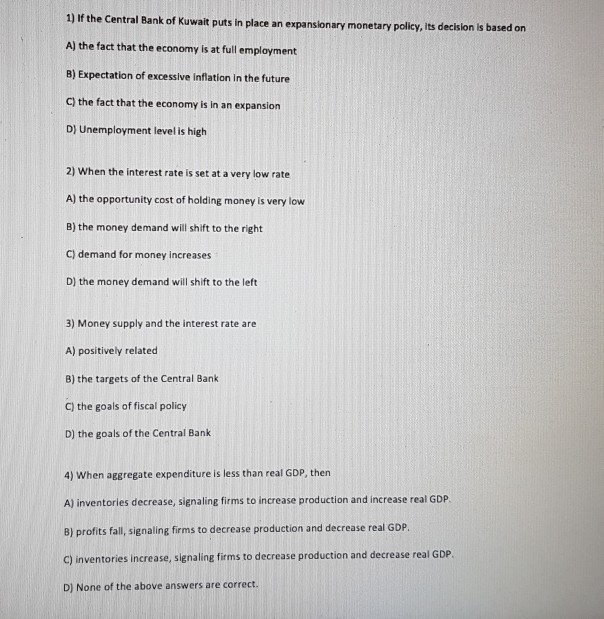Macroeconomic Multiple Choice Questions Answer All 10 Questions* 1) If the Central Bank of Kuwait puts in place an expansionary monetary policy, its decision is based on A) the fact that the economy is at full employment B) Expectation of excessive inflation in the future C) the fact that the economy is in an expansion D) Unemployment level is high 2) When the interest rate is set at a very low rate A) the opportunity cost of holding money is...

• ### 1) of the Central Bank of Kuwait puts in place an expansionary monetary policy, its decision...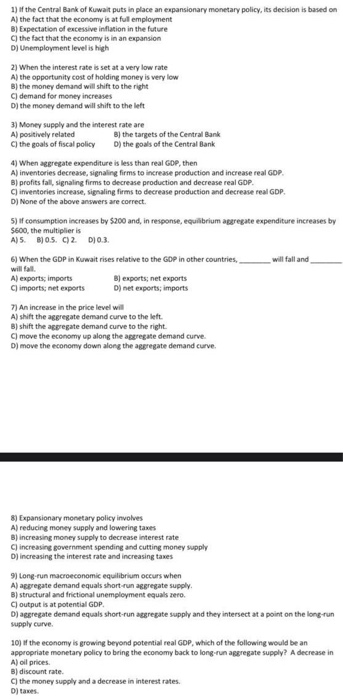1) of the Central Bank of Kuwait puts in place an expansionary monetary policy, its decision is based on A) the fact that the economy is at ful employment B) Expectation of excessive inflation in the future C) the fact that the economy is in an expansion D) Unemployment level is high 2) When the interest rate is set at a very low rate A) the opportunity cost of holding money is very low B) the money demand will shift...

• ### 1. In addition to the price level, what does the aggregate demand and aggregate supply model...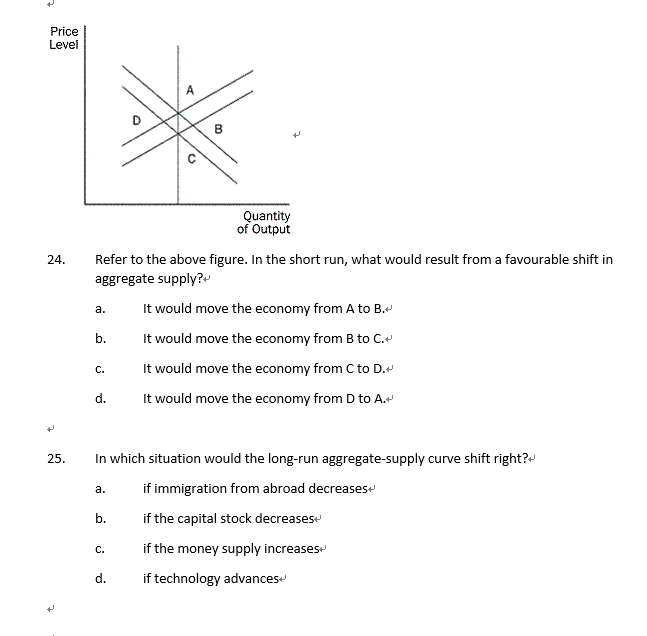1. In addition to the price level, what does the aggregate demand and aggregate supply model focus on?         a.    real GDP         b.    nominal GDP         c.     the real interest rate         d.    stock prices 2. Which statement best characterizes the long-run aggregate-supply curve?         a.    It is horizontal.         b.    It shows a positive relationship between price level and output.         c.     It demonstrates the importance of money in the economy....

• ### Question 89 An increase in the price level will ________. A. shift the aggregate demand curve...

Question 89 An increase in the price level will ________. A. shift the aggregate demand curve to the left B. shift the aggregate demand curve to the right C. move the economy up along a stationary aggregate demand curve D. move the economy down along a stationary aggregate demand curve BAM223 - PRINCIPLES OF ECONOMICS

• ### I. The economy of Zarland is operating below the full-employment level of output with a balanced budget. (a) Draw a correctly labeled graph of short-run aggregate supply, long-run aggregate supply, a...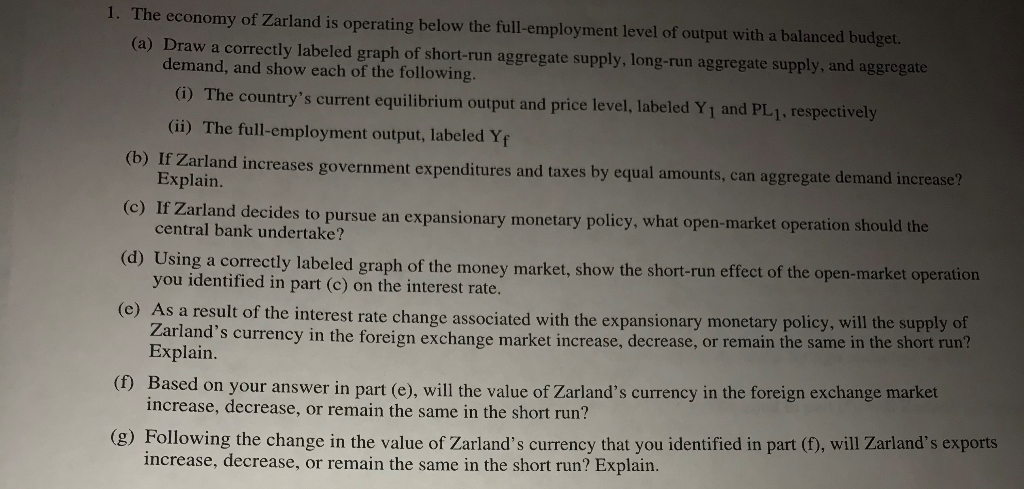I. The economy of Zarland is operating below the full-employment level of output with a balanced budget. (a) Draw a correctly labeled graph of short-run aggregate supply, long-run aggregate supply, and aggregate demand, and show each of the following. (Gi) The country's current equilibrium output and price level, labeled Yj and PL1. respectively (ii) The full-employment output, labeled Yf (b) Ir Zarland increases government expenditures and taxes by equal amounts, can aggregate demand increase? Explain. (c) If Zarland decides to...

• ### If at some specific interest rate the quantity of money demanded is less than the quantity...

If at some specific interest rate the quantity of money demanded is less than the quantity of money supplied, people will desire to buy interest-earning assets causing the interest rate to decrease. Select one: True False In recent years, the Fed has conducted policy by setting a target for the federal funds rate. Select one: True False A decrease in taxes is an expansionary fiscal policy designed to increase aggregate demand and reduce unemployment. Select one: True False If aggregate...

• ### 1. An above-full-employment equilibrium occurs when Group of answer choices aggregate demand decreases while neither the...

1. An above-full-employment equilibrium occurs when Group of answer choices aggregate demand decreases while neither the short-run nor long-run aggregate supply changes. short-run aggregate supply decreases while neither aggregate demand nor long-run aggregate supply changes. the equilibrium level of real GDP is greater than potential GDP. the equilibrium level of real GDP is less than potential GDP. 2. Which of the following shifts the aggregate demand curve rightward? Group of answer choices a decrease in consumption an increase in investment...

• ### Complete the sentences. Aggregate demand increases if expected future income, inflation, or profits And aggregate demand...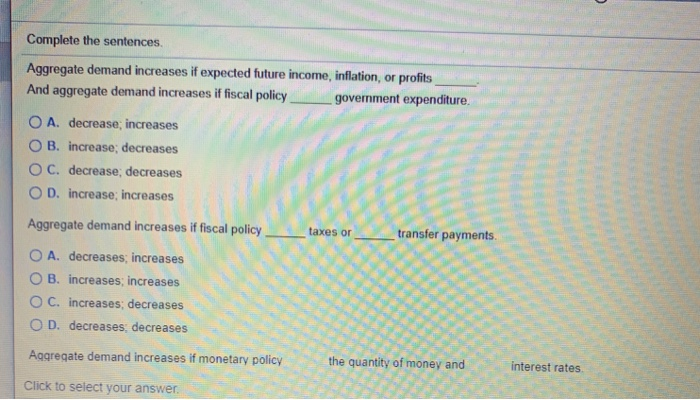Complete the sentences. Aggregate demand increases if expected future income, inflation, or profits And aggregate demand increases if fiscal policy government expenditure. SA O A. decrease; increases O B. increase; decreases OC. decrease, decreases OD. increase: increases Aggregate demand increases if fiscal policy transfer payments O A. decreases; increases OB. increases; increases O C. increases; decreases OD. decreases, decreases Aggregate demand increases if monetary policy the quantity of money and interest rates Click to select your answer. Complete the sentences....

• ### Question 1 An increase in the price level will ________ the real value of wealth and,...

Question 1 An increase in the price level will ________ the real value of wealth and, as a result, there will be ________ the aggregate demand curve. have no effect on; no change in increase; a rightward shift of reduce; an upward movement along reduce; a leftward shift of increase; an upward movement along 2. A severe drought hits a country and reduces farm output by 50 percent. This will impact aggregate demand. short-run aggregate supply and aggregate demand. short-run...